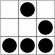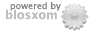"In het verleden behaalde resultaten bieden geen garanties voor de toekomst"

These are the ramblings of Matthijs Kooijman, concerning the software he hacks on, hobbies he has and occasionally his personal life.

Questions? Praise? Blame? Feel free to contact me.

My old blog (pre-2006) is also still available.

Sun Mon Tue Wed Thu Fri Sat

28 29 30 31
Tag Cloud&(With plugins: config, extensionless, hide, tagging, Markdown, macros, breadcrumbs, calendar, directorybrowse, entries_index, feedback, flavourdir, include, interpolate_fancy, listplugins, menu, pagetype, preview, seemore, storynum, storytitle, writeback_recent, moreentries)
Valid XHTML 1.0 Strict & CSS
Sendmail Horrors

As sysadmin of Inter-Actief, I have been working with our FreeBSD 6.0 webserver. After a bunch of permission and stability problems, everything seemed to be ok. We thought...

Now, somebody started complaining that the mails he had sent through this nice Joomla webinterface didn't arrive for half a day. We had some earlier problems, but that seemed to be just some incidental DNS failure. This time, about a dozen mails were stuk in our queue, due toe DNS failure. Since DNS was operating fine, something else was wrong

Diving into the wonderful world of sendmail (brr...) I found almost no useful documentation. After I while I found the "mailq" program that lists the queue. After disabling some security option somewhere, half the mails managed to get delivered: The most important half, which were in the "client mail queue". The mails in the "main mail queue" were still undelivered. What the hel is the difference anyway?

After venturing that enabling sendmail listening on our internet connection might solve stuff, there was the issue of trying that. Enabling the sendmailport and restarting sendmail didn't seem to fix it. Now, did it not work. But, didn't it work, or did sendmail just not get around to trying it again? If I could just force sendmail to retry everything, then I'd know for sure.

Right, after plowing down some configuration files, handbooks and manpages, the "sendmail -q" command seemed to do the trick. I think. Still no succes anyway... By the way, I was looking at the sendmail config files (I had to spend 10 minutes looking around in files and browsing the internet before I found the actual config file) and encountered the following marvelous snippet:

SU
R<$+> <$*> <$-$-> <$*>$: <$(access$4:$1$: ? $)> <$1> <$2> <$3 $4> <$5>
R<?> <$+> <$*> <+ $-> <$*>      $: <$(access $1$: ? $)> <$1> <$2> <+$3> <$4> R<?> <$+ + $* @> <$*> <$-$-> <$*>$: <$(access$5:$1+*@$: ? $)> <$1+$2@> <$3> <$4$5> <$6> R<?> <$+ + $* @> <$*> <+ $-> <$*>
$: <$(access $1+*@$: ? $)> <$1+$2@> <$3> <+ $4> <$5>
R<?> <$+ +$* @> <$*> <$- $-> <$*>
$: <$(access $5:$1@ $: ?$)> <$1+$2@> <$3> <$4 $5> <$6>
R<?> <$+ +$* @> <$*> <+$-> <$*>$: <$(access$1@ $: ?$)> <$1+$2@> <$3> <+$4> <$5> R<?> <$+> <$*> <$- $-> <$*>     $@ <$2> <$5> R<$+ <TMPF>> <$*> <$- $-> <$*>  $@ <<TMPF>> <$5>
R<$+> <$*> <$-$-> <$*>$@ <$1> <$5>


I am not sure what it is supposed to do, but I think this is supposed to the assembly form of brainfuck, that gets compiled to the actual sendmail program. Or something. Anyway, yikes! Oh and in case you were wondering, your layout is wrong, I did not add any spaces here, this is just how the configuration file looks....